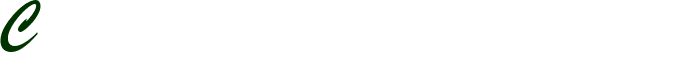# Derivatives and Limits

Derivatives and Limits

Description  Details

Discipline Mathematics

Assignment type : Math Assignment

Description

In this section of the project you are going to focus on using derivatives and limits, with some other mathematical ideas you already knew about before calculus. Your professor is going to give you two functions to work with. One of them will be a polynomial and the other from a family of functions that you are familiar with. You should follow this set of instructions for your first function and then go back and repeat the instructions for your second function.

Next, for each function identify the x-values where discontinuities occur. These should be identified as removable or non-removable. The intervals of continuity should then be shown, using the equation editor. Following that, identify any asymptotes that exist. Vertical asymptotes should be written as equations using the editor. Horizontal asymptotes should be written using limit notation and the editor. If asymptotes do not exist, that should be noted. After this, identify the end behavior of the function using the equation editor for formatting, if you have not done this with asymptotes already.

Now let’s focus on the first and second derivatives of each function. Create a complete graph of the first derivative and place it below the symbolic representation of the first derivative. Use the technology to determine the x and y intercepts of the first derivative. They should be labeled on the graph and identified below the symbolic representation of the respective first derivative function along with the titles of the axes, units, etc. that are part of the mentioned complete graph.

Then, create a complete graph of the second derivative and place it below the symbolic representation of the second derivative. Use the technology to determine the x and y intercepts of the second derivative. They should be labeled on the graph and identified below the symbolic representation of the respective second derivative function along with the titles of the axes, units, etc. that are part of the mentioned complete graph.

Finally, you should create a complete graph that shows the function and its two derivatives on the same set of axes. Again, do this for each function. (You should have a total of six graphs in your report after this instruction… graphs of the two original functions, graphs of the first derivatives of each function, and graphs of the second derivatives of each function.) Using this graph as a basis, discuss the relationships between the three graphs. Discuss how the intercepts on the graphs of derivatives are related to the graphs of their respective functions. Similarly, discuss how the values of the derivatives are related to the behavior of the original function.

 MathType, which interfaces with MicroSoft Word and other word processing packages is available on many of the computers on campus. Also, MicroSoft Word and Google Docs have a built in equation editor that you can use to format your equations / functions. In MicroSoft Word just choose Insert from the top menu bar and then Equation.

 A complete graph means that the axes are labeled with their respective variables; the axes should also have titles with units; the X and Y intercepts should be labeled; the equation of the graph should be labeled; and graph should have a title. Remember a graph tells a story and these labels help to tell that story.

 A complete graph means that the axes are labeled with their respective variables; the axes should also have titles with units; the X and Y intercepts should be labeled; the equation of the graph should be labeled; and graph should have a title. Remember a graph tells a story and these labels help to tell that story.

 A complete graph means that the axes are labeled with their respective variables; the axes should also have titles with units; the X and Y intercepts should be labeled; the equation of the graph should be labeled; and graph should have a title. Remember a graph tells a story and these labels help to tell that story.

 A complete graph means that the axes are labeled with their respective variables; the axes should also have titles with units; the X and Y intercepts should be labeled; the graph itself should be labeled; and graph should have a title. Remember a graph tells a story and these labels help to tell that story.

Format MLA

Volume of 825 – 1650 pages (5 pages)

Type of service: Editing## You Need a Professional Writer To Work On Your Paper?## Do you have an Urgent Assignment?;
BestQualityExperts
X
error:

Disclaimer: The services that we offer are meant to help the buyer by providing a guideline.
The product provided is intended to be used for research or study purposes only.